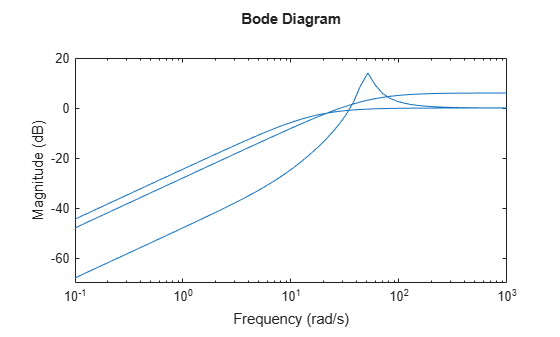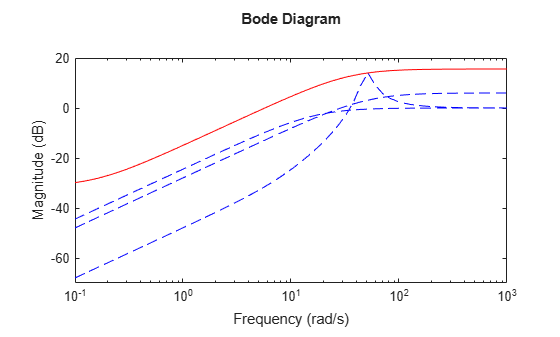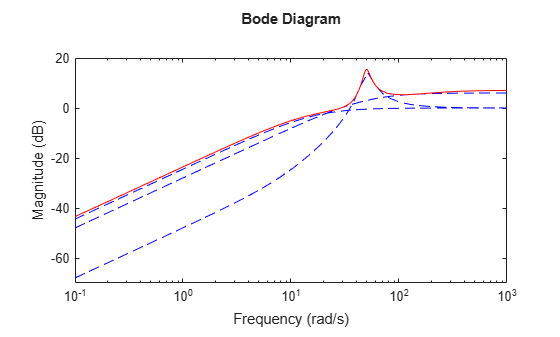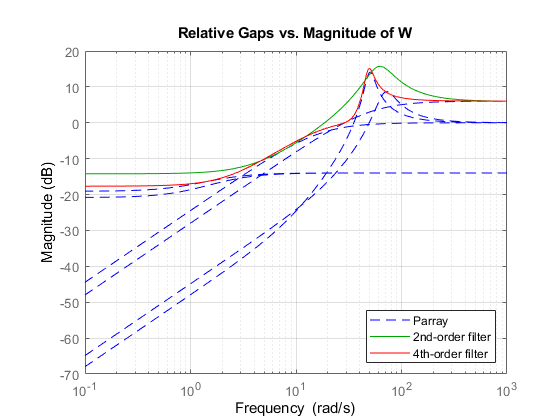ucover

Fit uncertain model to set of LTI responses

Description

example

usys = ucover(Parray,Pnom,ord) returns an uncertain model usys with nominal value Pnom and whose range of behaviors includes all responses in the LTI array Parray. The uncertain model structure is of the form $usys=Pnom\left(I+W\left(s\right)\Delta \left(s\right)\right)$, where:

• Δ is a ultidyn object that represents uncertain dynamics with unit peak gain.

• W is a stable, minimum-phase shaping filter of order ord that adjusts the amount of uncertainty at each frequency. For a MIMO Pnom, W is diagonal, with the orders of the diagonal elements given by ord.

usys = ucover(Parray,Pnom,ord1,ord2,utype) returns an uncertain model with the structure specified by utype.

• utype = 'InputMult' — Input multiplicative form, in which usys = Pnom*(I + W1*Delta*W2)

• utype = 'OutputMult' — Output multiplicative form, in which usys = (I + W1*Delta*W2)*Pnom

• utype = 'Additive' — Additive form, in which usys = Pnom + W1*Delta*W2

Delta represents uncertain dynamics with unit peak gain, and W1 and W2 are diagonal, stable, minimum-phase shaping filters with orders specified by ord1 and ord2, respectively.

[usys,info] = ucover(Parray,___) returns a structure info that contains information about the fit. You can use this syntax with any of the previous input-argument combinations.

[usys,info] = ucover(Pnom,info_in,ord1,ord2) improves the fit using initial filter values in the info result. Supply new orders ord1 and ord1 for W1 and W2. When you are trying different filter orders to improve the result, this syntax speeds up iteration by letting you reuse previously computed information.

Examples

collapse all

Fit an uncertain model to an array of LTI responses. The responses can be, for example, the results of multiple runs to acquire frequency response data from a physical system.

For this example, generate the frequency response data by creating an array of LTI models and sampling the frequency response of those models.

Pnom = tf(2,[1 -2]);
p1 = Pnom*tf(1,[.06 1]);
p2 = Pnom*tf([-.02 1],[.02 1]);
p3 = Pnom*tf(50^2,[1 2*.1*50 50^2]);
array = stack(1,p1,p2,p3);
Parray = frd(array,logspace(-1,3,60));

The frequency response data in Parray represents three separate data acquisition experiments on the system.

Plot the relative errors between the nominal plant response and the three models in the LTI array.

relerr = (Pnom-Parray)/Pnom;
bodemag(relerr)If you use a multiplicative uncertainty model structure, you want the magnitude of the shaping filter to fit the maximum relative error at each frequency. Use this requirement to help choose the order of the shaping filter. First, try a first-order shaping filter.

[P,Info] = ucover(Parray,Pnom,1);

P is an uncertain state-space (uss) model that captures the uncertainty as a ultidyn uncertain dynamics block.

P.Uncertainty
ans = struct with fields:
Parray_InputMultDelta: [1x1 ultidyn]

The Info structure contains other information about the fit, including the resulting shaping filter, Info.W1. Plot the response to see how well the shaping filter fits the relative errors.

W = Info.W1;
bodemag(relerr,'b--',W,'r',{0.1,1000});The plot shows that the filter W is too conservative and exceeds the maximum relative error at most frequencies. To obtain a tighter fit, rerun the function using a fourth-order filter.

[P,Info] = ucover(Parray,Pnom,4);

Evaluate the fit by plotting the Bode magnitude plot.

W = Info.W1;
bodemag(relerr,'b--',W,'r',{0.1,1000});This plot shows that for the fourth-order filter, the magnitude of W closely matches the largest error, yielding the minimum uncertainty that captures all the variation.

Input Arguments

collapse all

Array of models to cover with a dynamically uncertain model, specified as an array of LTI models such as tf, ss, zpk, or frd models.

Nominal model of the uncertain model, specified as an LTI model such as a tf, ss, zpk, or frd model.

Filter order, specified as an integer, vector, or []. The values in ord specify the number of states of each diagonal entry of the shaping filter W. Specify ord as:

• A single integer, for a SISO Pnom, or to use a scalar filter W for a MIMO Pnom.

• A vector of length equal to the number of outputs in Pnom, to specify different orders for each diagonal entry of W.

• [], to set W = 1.

Filter orders, specified as integers, vectors, or []. The values in ord1 and ord2 specify the number of states of each diagonal entry of the shaping filters W1, and W2, respectively. Specify ord1 and ord2 as:

• A single integer, to use scalar filters for W1 and W2.

• A vector, to specify different orders for each diagonal entry of W1 and W2. The lengths of these vectors depend on the uncertainty model you specify in utype. The following table gives the lengths, where Pnom has Nu inputs and Ny outputs.

utypelength(ord1)length(ord2)
'InputMult'NuNu
'OutputMult'NyNy
• [], to set W1 = 1 or W2 = 1.

Uncertainty model, specified as one of the following.

• 'InputMult' — Input multiplicative form, in which usys = Pnom*(I + W1*Delta*W2)

• 'OutputMult' — Output multiplicative form, in which usys = (I + W1*Delta*W2)*Pnom

• 'Additive' — Additive form, in which usys = Pnom + W1*Delta*W2

Delta represents uncertain dynamics with unit peak gain, and W1 and W2 are diagonal, stable, minimum-phase shaping filters with orders specified by ord1 and ord2, respectively.

Use additive uncertainty to model the absolute gaps between Pnom and Parray, and multiplicative uncertainty to model relative gaps.

For SISO models, input and output multiplicative uncertainty are equivalent. For MIMO systems with more outputs than inputs, the input multiplicative structure might be too restrictive and might not adequately cover the range of models.

Details from a previous ucover run, specified as a structure generated as the info output of the previous run. Use this input when calling ucover iteratively to improve fit results by trying different filter orders.

Output Arguments

collapse all

Uncertain model, returned as a uss or ufrd model. The returned model is a uss model, unless Parray or Pnom are frequency-response data (frd) models, in which case usys is a ufrd model.

usys has one uncertain element, a ultidyn block with the name given in the DeltaName field of the info output argument.

Information about the fit, returned as a structure containing the following fields.

FieldValue
W1

Fitted shaping filter W or W1, returned as a state-space (ss) model.

W2

Fitted shaping filter W2, returned as a state-space (ss) model.

W1opt

W or W1 evaluated on a frequency grid, returned as an frd model.

W2opt

W2 evaluated on a frequency grid, returned as an frd model.

Ord1

Orders of the diagonal elements of W or W1, returned as a scalar or vector. These values are the values you supply with the ord or ord1 input argument.

Ord2

Orders of the diagonal elements of W2, returned as a scalar or vector. These values are the values you supply with the ord2 input argument.

Type

Uncertainty model used for the fit, returned as 'InputMult', 'OutputMult', or 'Additive'.

DeltaName

Name of the ultidyn block of usys that represents the uncertainty model Delta, returned as a character vector.

Residual

Residuals of the fit, returned as an array of frd models with the same array dimensions as Parray.

Algorithms

ucover fits the responses of LTI models in Parray by modeling the gaps between Parray and the nominal response Pnom as uncertainty on the system dynamics. To model the frequency distribution of these unmodeled dynamics, ucover measures the gap between Pnom and Parray at each frequency on a grid, and selects shaping filters whose magnitude approximates the maximum gap.

To design the minimum-phase shaping filters W1 and W2, the ucover command performs two steps:

1. Compute the optimal values of W1 and W2 on a frequency grid.

2. Fit W1 and W2 values with the dynamic filters of the specified orders using fitmagfrd.

The model structure $usys=Pnom\left(I+W\left(s\right)\Delta \left(s\right)\right)$ that you obtain using usys = ucover(Parray,Pnom,ord) corresponds to W1 = W and W2 = 1.

For instance, the following figure shows the relative gap between the nominal response and six LTI responses, enveloped using a second-order shaping filter and a fourth-order filter.If you use the single-filter syntax usys = ucover(Parray,Pnom,ord), the software sets the uncertainty to W*Delta, where Delta is a ultidyn object that represents unit-gain uncertain dynamics. Therefore, the amount of uncertainty at each frequency is specified by the magnitude of W and closely tracks the gap between Pnom and Parray. In the above figure, the fourth-order filter tracks the maximum gap more closely and therefore yields a less conservative estimate of uncertainty.